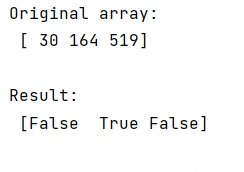# Easy way to test if each element in a numpy array lies between two values?

Given a NumPy array, we have to check if each element in it lies between two values.
Submitted by Pranit Sharma, on January 19, 2023

NumPy is an abbreviated form of Numerical Python. It is used for different types of scientific operations in python. Numpy is a vast library in python which is used for almost every kind of scientific or mathematical operation. It is itself an array which is a collection of various methods and functions for processing the arrays.

## Checking whether each element in a numpy array lies between two values

Suppose that we are given a NumPy array that contains some integer values. We need to check that each value of this array lies between two values (say 50 and 100).

For this purpose, we can simply write our condition on our array and use the logical AND operation between both conditions. It will return True if both the conditions are True and False if any of them is False.

Let us understand with the help of an example,

## Python code to check if each element in a numpy array lies between two values

```# Import numpy
import numpy as np

# Creating an array
arr = np.arange(10)

# Display original array
print("Original Array:\n",arr,"\n")

# Shuffling array
np.random.shuffle(arr)

# Display result
print("Shuffled array:\n",arr,"\n")
```

Output:What's New (MCQs)

Top Interview Coding Problems/Challenges!

IncludeHelp's Blogs

Languages: » C » C++ » C++ STL » Java » Data Structure » C#.Net » Android » Kotlin » SQL
Web Technologies: » PHP » Python » JavaScript » CSS » Ajax » Node.js » Web programming/HTML
Solved programs: » C » C++ » DS » Java » C#
Aptitude que. & ans.: » C » C++ » Java » DBMS
Interview que. & ans.: » C » Embedded C » Java » SEO » HR
CS Subjects: » CS Basics » O.S. » Networks » DBMS » Embedded Systems » Cloud Computing
» Machine learning » CS Organizations » Linux » DOS
More: » Articles » Puzzles » News/Updates